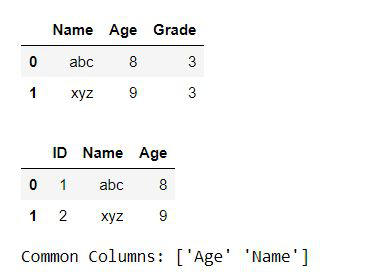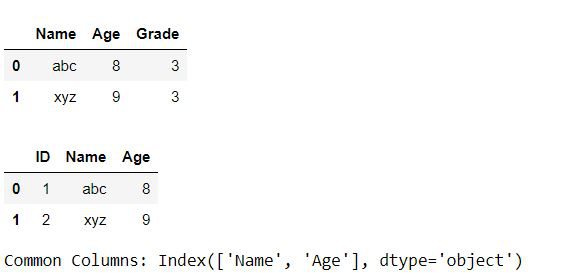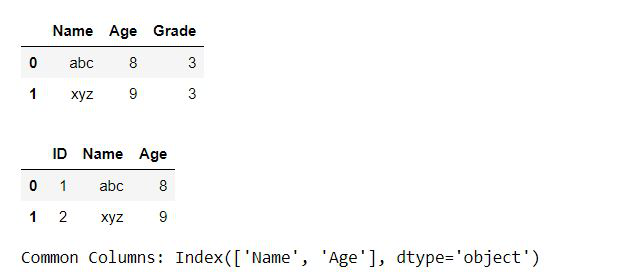Related Articles
Find Columns Shared By Two Data Frames
• Last Updated : 11 Dec, 2020

Pandas is the open-source Python library used for Data analysis and manipulation. It is fast, powerful, flexible, and easy to use. In this article, we will discuss how to find columns that are common between two Data Frames. Below is the different approach that can be used to find common columns.

Method 1: Using Numpy intersect1d method

In this example, we will create Pandas Dataframe from the list, and then we will use Numpy’s intersect1d() method which will return columns that are common between two Dataframes.

## Python3

 `# Importing libraries``import` `pandas as pd``import` `numpy as np`` ` `# Creating Dataframes``a ``=` `[{``'Name'``: ``'abc'``, ``'Age'``: ``8``, ``'Grade'``: ``3``},{``'Name'``: ``'xyz'``, ``'Age'``: ``9``, ``'Grade'``: ``3``}]``df1 ``=` `pd.DataFrame(a)``b ``=` `[{``'ID'``: ``1``,``'Name'``: ``'abc'``, ``'Age'``: ``8``},{``'ID'``: ``2``,``'Name'``: ``'xyz'``, ``'Age'``: ``9``}]``df2 ``=` `pd.DataFrame(b)`` ` `# Pring Dataframes``display(df1)``display(df2)`` ` `# Finding Common columns``a ``=` `np.intersect1d(df2.columns, df1.columns)`` ` `# Printing common columns``print` `(``"Common Columns:"``,a)`

Output:Method 2: Using Pandas intersection method

In this example, we will create Pandas Dataframe from the list, and then we will use Panda’s intersection() method which will return columns that are common between two Dataframes.

## Python3

 `# Importing libraries``import` `pandas as pd`` ` `# Creating Dataframes``a ``=` `[{``'Name'``: ``'abc'``, ``'Age'``: ``8``, ``'Grade'``: ``3``},``     ``{``'Name'``: ``'xyz'``, ``'Age'``: ``9``, ``'Grade'``: ``3``}]`` ` `df1 ``=` `pd.DataFrame(a)``b ``=` `[{``'ID'``: ``1``,``'Name'``: ``'abc'``, ``'Age'``: ``8``},``     ``{``'ID'``: ``2``,``'Name'``: ``'xyz'``, ``'Age'``: ``9``}]`` ` `df2 ``=` `pd.DataFrame(b)`` ` `# Pring Dataframes``display(df1)``display(df2)`` ` `# Finding Common columns``a ``=` `df2.columns.intersection(df1.columns)`` ` `# Printing common columns``print` `(``"Common Columns:"``,a)`

Output:Method 3: In this example, we will use & operator to find common columns.

## Python3

 `# Importing libraries``import` `pandas as pd`` ` `# Creating Dataframes``a ``=` `[{``'Name'``: ``'abc'``, ``'Age'``: ``8``, ``'Grade'``: ``3``},``     ``{``'Name'``: ``'xyz'``, ``'Age'``: ``9``, ``'Grade'``: ``3``}]`` ` `df1 ``=` `pd.DataFrame(a)``b ``=` `[{``'ID'``: ``1``,``'Name'``: ``'abc'``, ``'Age'``: ``8``},``     ``{``'ID'``: ``2``,``'Name'``: ``'xyz'``, ``'Age'``: ``9``}]`` ` `df2 ``=` `pd.DataFrame(b)`` ` `# printing Dataframes``display(df1)``display(df2)`` ` `# Finding Common columns``a ``=` `df1.columns & df2.columns`` ` `# Printing common columns``print` `(``"Common Columns:"``,a)`

Output:Attention geek! Strengthen your foundations with the Python Programming Foundation Course and learn the basics.

To begin with, your interview preparations Enhance your Data Structures concepts with the Python DS Course.

My Personal Notes arrow_drop_up